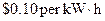Chapter 34, Problem 31PE

Chapter
Section
Textbook Problem

A section of superconducting wire carries a current of 100 A and requires 1.00 L of liquid nitrogen per hour to keep it below its critical temperature. For it to be economically advantageous to use a superconducting wire, the cost at cooling the wire must be less than the cost of energy lost to heat in the wire. Assume that the cost of liquid nitrogen is $0.30 per liter, and that electric energy costsWhat is the resistance of a normal wire that costs as much in wasted electric energy as the cost of liquid nitrogen for the superconductor? To determine The resistance of the normal wire having the cost in wasted of electrical energy as the liquid nitrogen for the superconductor. Explanation Given: Current across the superconducting wire, I=100A Formula: Formula to calculate the resistance of the normal wire is, E=I2Rt ......... (I) Where, • I is the current flowing across the superconducting wire. • R is the resistance of the normal wire. • t is the time. Calculation: Since, 1L of nitrogen is required per hour. The electrical energy cost is$0.10 per kWh and the cost of liquid nitrogen is \$0.30 per liter. So, the total energy waste is 3kW.

Rearrange equation (I) to calculate the resistance

Still sussing out bartleby?

Check out a sample textbook solution.

See a sample solution

The Solution to Your Study Problems

Bartleby provides explanations to thousands of textbook problems written by our experts, many with advanced degrees!

Get Started

Find more solutions based on key concepts